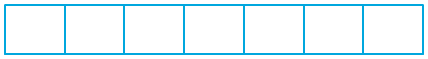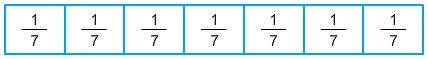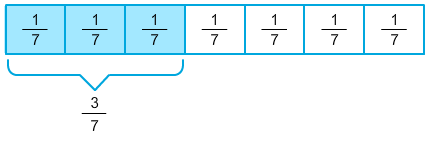# Decompose Fractions into Unit Fractions

Rate 0 stars
Quiz size:
Message preview:
Someone you know has shared quiz with you:

To play this quiz, click on the link below:

https://www.turtlediary.com/quiz/decompose-fractions-into-unit-fractions.html

Hope you have a good experience with this site and recommend to your friends too.

Login to rate activities and track progress.
Login to rate activities and track progress.

A unit fraction is a fraction in which numerator is 1.

Let's decompose
 3 7
as a sum of unit fractions .

Make a model to identify the sum of the unit fractions.This model represents a whole divided into 7 equal parts.

Here, each part represents the unit fraction,
 1 7
.Now, select 3 parts to represent the fraction
 3 7
.So,
 3 7
=
 1 7
+
 1 7
+
 1 7
.

ds

A B C D E F G H I J K L M N O P Q R S T U V W X Y Z

### Help

##### Remember :

The smallest number is the one that comes first while counting.

##### Solution :

To arrange the given numbers in order from smallest to greatest, find the smallest number among all the given numbers.

21,27,23

21 is the smallest number.